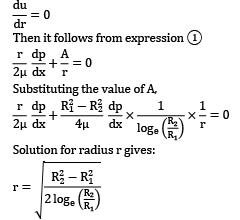Courses

# Test: Viscous Flow of Incompressible Fluids, Flow Losses Level - 3

## 20 Questions MCQ Test Engineering Mechanics | Test: Viscous Flow of Incompressible Fluids, Flow Losses Level - 3

Description
This mock test of Test: Viscous Flow of Incompressible Fluids, Flow Losses Level - 3 for Civil Engineering (CE) helps you for every Civil Engineering (CE) entrance exam. This contains 20 Multiple Choice Questions for Civil Engineering (CE) Test: Viscous Flow of Incompressible Fluids, Flow Losses Level - 3 (mcq) to study with solutions a complete question bank. The solved questions answers in this Test: Viscous Flow of Incompressible Fluids, Flow Losses Level - 3 quiz give you a good mix of easy questions and tough questions. Civil Engineering (CE) students definitely take this Test: Viscous Flow of Incompressible Fluids, Flow Losses Level - 3 exercise for a better result in the exam. You can find other Test: Viscous Flow of Incompressible Fluids, Flow Losses Level - 3 extra questions, long questions & short questions for Civil Engineering (CE) on EduRev as well by searching above.
QUESTION: 1

### For a conical bearing, 100 watt power gets dissipated when a shaft with maximum cone radius 10 cm turns with 600 rpm over a uniform fluid layer of thickness 0.1 cm. If semi-angle for the conical bearing is 30°, make calculations for the dynamic viscosity of the fluid (in SI units).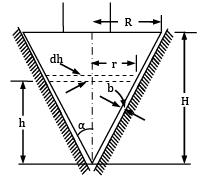Solution: Consider an elementary ring of bearing surface of radius r and thickness dh at a distance h from the cone apex. Then r = h tan α and bearing area of the elementary ring equals dA = 2πr (dh sec α)

= 2πh tan α dh sec α

Viscous shear stress,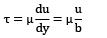Since the shaft has an angular velocity ω, The tangential velocity, u = ωr = ωh tan α

∴ Viscous shear stress,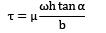Tangential resistance on the ring, dF = shear stress × area of the ring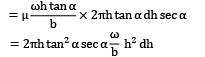Torque = moment of tangential resistance = dF × r = dF × h tan α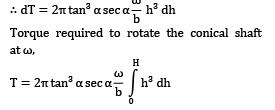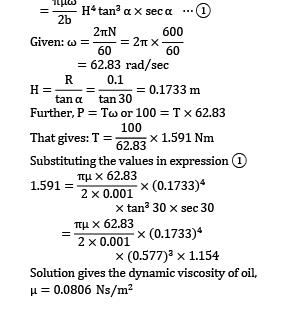QUESTION: 2

### Air (T = 20°C and p = 101kPa absolute) flows at a rate of 2.5 m3⁄s in a horizontal, commercial steel, HVAC duct of 0.3 m × 0.6 m. (Note that HVAC is an acronym for heating, ventilating, and air conditioning.) What is the pressure drop in mm of water per 50 m of duct? (Darcy friction factor, f = 0.015)

Solution: 1. Pressure drop due to head loss (hL)

p1 − p2 = ρghL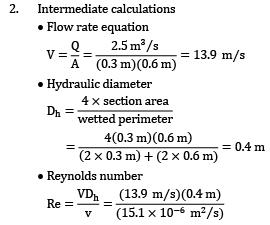= 368,000

Thus, flow is turbulent. · Resistance coefficient: f = 0.015

3. Darcy-Weisbach equation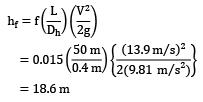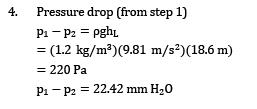QUESTION: 3

### Water at 10°C flows from a large reservoir to a smaller one through a 5-cm-diameter cast iron piping system, as shown in Fig. Determine the elevation z1 (in m) for a flow rate of 6 L/s.The density and dynamic viscosity of water at 10° C are ρ = 999.7 kg⁄m3 and μ = 1.307 × 10−3 kg⁄m ∙ s. The coefficient of friction = 0.0315.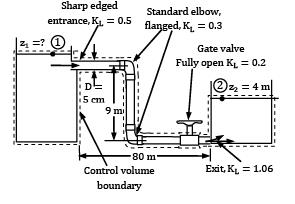Solution: The density and dynamic viscosity of water at 10° C are ρ = 999.7 kg⁄m3 and μ = 1.307 × 10−3 kg⁄m ∙ s.

The piping system involves 89 m of piping, a sharp-edged entrance (KL = 0.5), two standard flanged elbows (KL = 0.3 each), a fully open gate valve (KL = 0.2), and a submerged exit (KL = 1.06). We choose points 1 and 2 at the free surfaces of the two reservoirs. Noting that the fluid at both points is open to the atmosphere (and thus p1 = p2 = patm ) and that the fluid velocities at both points are zero (V1 = V2 = 0), the energy equation for a control volume between these two points simplifies to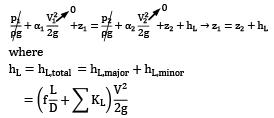Since the diameter of the piping system is constant. The average velocity in the pipe and the Reynolds number are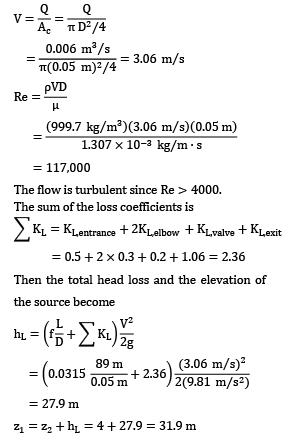Therefore, the free surface of the first reservoir must be 31.9 m above the ground level to ensure water flow between the two reservoirs at the specified rate.

QUESTION: 4

Oil having a specific gravity of 0.8 and a viscosity of 2 × 10−2 N ∙ s/m2 flows downward between two vertical smooth plates spaced 10 mm apart. If the discharge per meter of width is 0.01 m2⁄s, what is the pressure gradient dp⁄ds for this flow? Give your answer in kPa/m.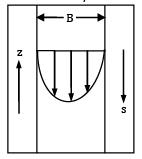Solution: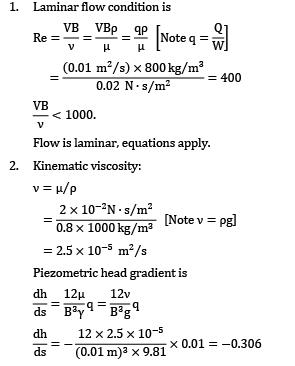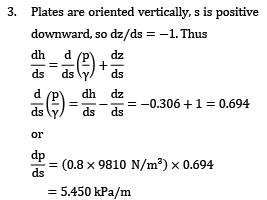Note: The pressure increases in the downward direction which means that the pressure, in part, supports the weight of the fluid.

QUESTION: 5

Two reservoirs open to the atmosphere are connected by a pipe 800 metres long. The pipe goes over a hill whose height is 6 m above the level of water in the upper reservoir. The pipe diameter is 300 mm and friction factor f = 0.032. The difference in water levels in the two reservoirs is 12.5 m. If the absolute pressure of water anywhere in the pipe is not allowed to fall below 1.2 m of water in order to prevent vapour formation, calculate the length of pipe in the portion between the upper reservoir and the hill summit. Neglect bend losses.

Solution: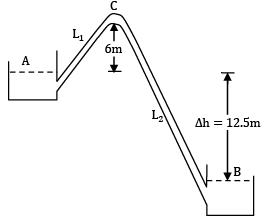Let the length of pipe upstream of C be L1 and that of the downstream be L2 It is given L1 + L2 = 800 m Considering the entry, friction and exit losses, The total loss from A to C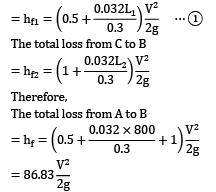Applying Bernoulli’s equation between ! and B, we have

Δ H = hf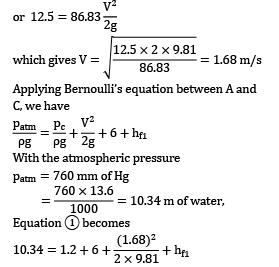which gives hf1 = 2.99 m Using the value of hf1 = 2.99 m, and V = 1.68 m/s in equation ① we get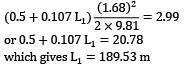QUESTION: 6

Water (T = 20°C) flows from a tank through a 50 cm diameter steel pipe. Determine the discharge of water in m3/s. (Friction factor = 0.012)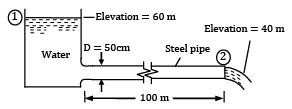Properties: 1. Water at 20°C, ν = 1 × 10−6 m2⁄s 2. The Darcy friction factor = 0.012 Also consider all possible losses in the flow.

Solution: All the possible losses will be major head loss and minor head loss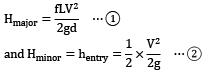Note: Since water is flowing out into the atmosphere from ②; there will be no exit losses as exit loss occurs only when fluid flows from a pipe to a tank. Applying Bernoulli’s equation at ① and ②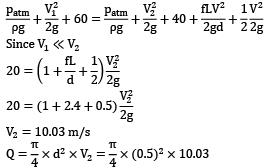Q = 1.96 m3/s

QUESTION: 7

Water ρ = 1000 kg/m3and ν = 1.02 × 10−6 m2/s is pumped between two reservoirs at 5.66 × 10−2 m3/s through 122 m of 5.1 cm diameter pipe and several minor losses, as shown in Fig. Compute the pump power required in kW. f = 0.0216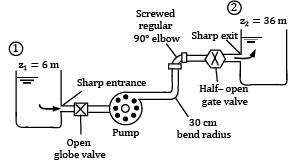Loss K Sharp entranceOpen globe valve30 cm bendRegular 90° elbowHalf-closed gate valveSharp exit 0.56.90.250.952.71.0

Solution: Writing the steady flow energy equation between sections 1 and 2, the two reservoir surfaces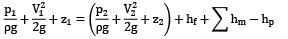where hp is the head increase across the pump. But since p1 = p2 and V1 = V2 ≈ 0, solving for the pump head: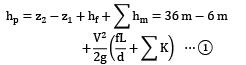Now with the flow rate known, calculate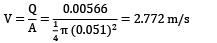Now list and sum the minor loss coefficients: The Reynolds number is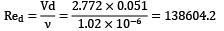f = 0.0216. Substitute into Equation ①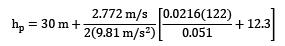= 30 m + 25 m = 55 m Pump head

The pump must provide a power to the water of

P = ρgQhp = 1000 × 9.81 × 0.00566 × 55 = 3.054 kW

QUESTION: 8

An oil with a viscosity of μ = 0.40 N ∙ s⁄m2 and density ρ = 900 kg⁄m3 flows in a pipe of diameter D = 0.020 m.

(i) What pressure drop, p1 − p2 , is needed to produce a flow rate of Q = 2.0 × 10−5 m3⁄s if the pipe is horizontal with x1 = 0 and x2 = 10 m?

(ii) How steep a hill, θ must the pipe be on if the oil is to flow through the pipe at the same rate as in part (a), but with p1 = p2?

Solution: (a) If the Reynolds number is less than 2100 the flow is laminar and the equations derived in this section are valid. since the average velocity is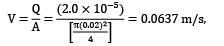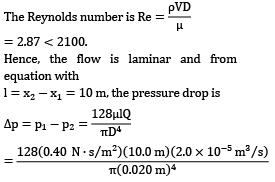or Δp = 20,400 N/m2 = 20.4 kPa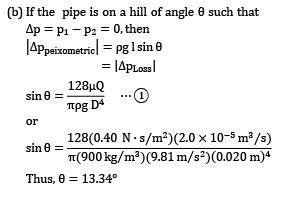QUESTION: 9

A sudden enlargement of a water pipeline from a diameter of 0.24 m to 0.48 m. The HGL rises by 10 mm. Then find the discharge in m3/s.

Solution: From Bernoulli’s equation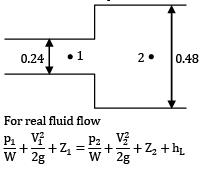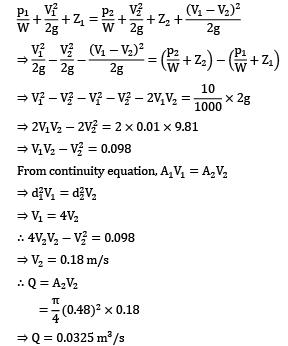QUESTION: 10

Three reservoirs A, B and C are connected by a pipe system as shown in figure. The height of water level in reservoir C is __________ m. Take f = 0.024 for all pipes.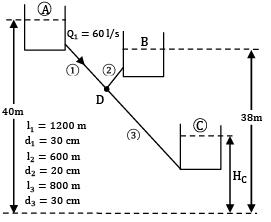Solution: Applying Bernoulli’s equation between ! and D, we have,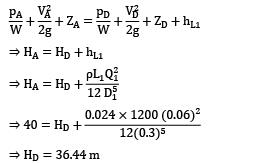Hence HB > HD; hence flow will be from B to D Applying Bernoulli’s equation between, B and D we have,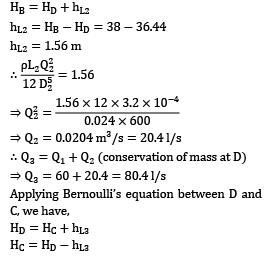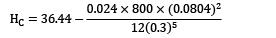⇒ HC = 36.44 − 4.26

⇒ HC = 32.2 m

QUESTION: 11

Air flowing into a 2-ft–square duct with a uniform velocity of 10 ft/s forms a boundary layer on the walls as shown in Fig. The fluid within the core region (outside the boundary layers) flows as if it were inviscid. From advanced calculations it is determined that for this flow the boundary layer displacement thickness is given by δ∗ = 0.0070(x)1⁄2 where δ∗ and x are in feet. Determine the velocity U = U(x) of the air within the duct but outside of the boundary layer.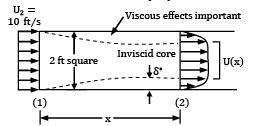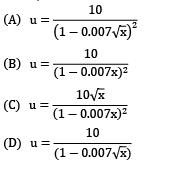Solution: If we assume incompressible flow (a reasonable assumption because of the low velocities involved), it follows that the volume flowrate across any section of the duct is equal to that at the entrance (i. e. , Q1 = Q2). That is,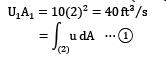According to the definition of the displacement thickness, δ, the flowrate across section (2) is the same as that for a uniform flow with velocity U through a duct whose walls have been moved inward by δ. That is,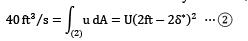By combining Equations (1) and (2) we obtain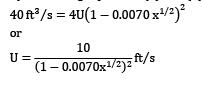QUESTION: 12

A container full of oil has a horizontal parallel crack in its end wall which is 500 mm wide and 50 mm thick in the direction of flow. The pressure difference between two faces of the crack is 10 kPa and the crack forms a gap of 0.4 mm between the parallel surfaces.

Calculate

(i) Maximum leakage velocity;

(ii) Shear stress at the boundary.

Take specific gravity and viscosity of oil equal to 0.85 and 1.8 poise respectively.

Solution: μ = 1.8 poise = 1.8 × 0.1 = 0.18 Ns⁄m2

b = 0.4 mm = 0.0004 m

l = 50 mm = 0.05 m

p1 − p2 = 10 kPa = 10 × 103 N⁄m2

(i) The oil leakage through the crack corresponds to the case of flow between two stationary parallel surfaces for which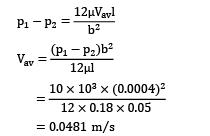(ii) The maximum velocity occurs midway between the crack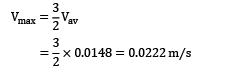Shear stress at the wall,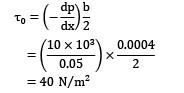QUESTION: 13

A spindle 5 cm in diameter turns 110 revolutions per minute in a bearing 5.004 cm internal diameter. The intervening space is filled to 20 cm depth with a lubricating oil of dynamic viscosity 0.08 Pa‑s. If the oil pressure is 4000 kPa, make calculations for the oil leakage along the spindle and the resisting torque due to viscous drag of the oil film.

Solution: Given μ = 0.08 Pa‑s = 0.08 Ns⁄m2 radial clearance,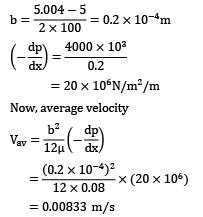∴ Oil leakage = clearance area × average velocity

= π db × Vav = π × 0.05 × 0.2 × 10−4 × 0.00833 = 2.61 × 10−8 m3⁄s

Shear stress,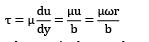where ω is the angular velocity and r is the radius of the spindle. Viscous torque

= viscous force × radial distance

= (shear stress × area) × radiusQUESTION: 14

Determine the amount of flow (in m3 /s) per meter width between two parallel plates when one is moving relative to the other with a velocity of 3 m/s in the negative x-direction, if dp /dx = −100 N⁄cm2 /cm, μ is 0.4 poise and the distance between the plates is 1 mm.

Solution: For laminar flow between parallel plates having relative motion (lower plate fixed and the upper plate moving uniformly with velocity V), the velocity distribution is given by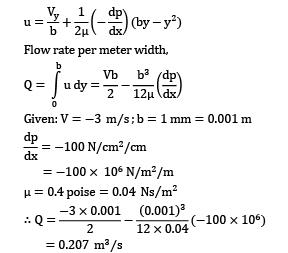The discharge is in the positive x-direction, i.e., in the direction opposite to that of moving plate.

QUESTION: 15

Laminar flow of a fluid of viscosity 0.90 kg⁄ms and mass density 1250 kg⁄m3 occurs between a pair of plates of extensive width; the plates are 10 mm apart and are inclined at 45 degree to the horizontal. Pressure gauges mounted at two points 1.2 m vertically apart on the upper plate record pressure of 75 kN⁄m2 and 250 kN⁄m2 at the lower and upper point respectively. The upper plate moves with a velocity of 2.0 m⁄s relative to the lower plate but in a direction opposite the fluid flow. Make calculations for (i) the maximum flow velocity, and (ii) the shear stress on the upper plate.

Solution: Since the plates are placed uniformly apart, velocity head would be the same at the two sections and as such flow direction will be dictated by the value of the piezometric head.

Taking a horizontal line passing through point 2 as datum Piezometric head at point 1,

p1 = p1 + wy1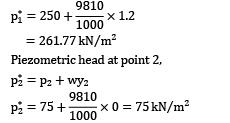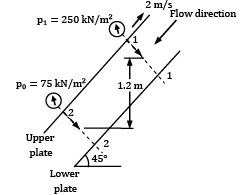Since p1 is greater that p2 , the direction of flow is from section 1-1 to section 2-2, i.e., downward. Apparently the upper plate is moved up the slope. Pressure gradient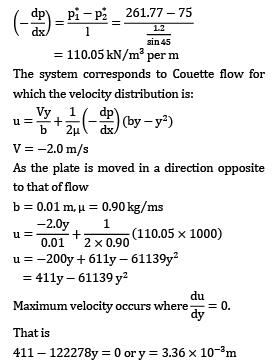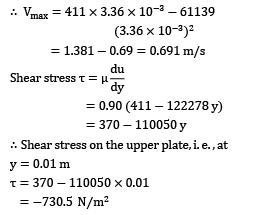QUESTION: 16

Two reservoirs are connected through a 300 mm diameter pipe line, 1000 m long as shown in figure. At a point B, 300 m from reservoir A, a valve is inserted on a short branch line which discharges to the atmosphere. The valve may be regarded as a rounded orifice 75 mm diameter, Cd = 0.65. If friction factor f for all the pipes is 0.013. Estimate the leakage through the short pipe line at B (in m3/s).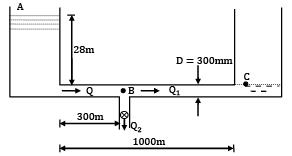Solution: Let the flow rate through the first 300 m of the pipe be Q and the flow rates through the next 700 m of the pipe and the short branch line containing the valve be Q1 and Q2 respectively.

From continuity, Q = Q1 + Q2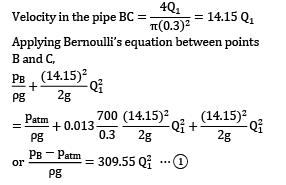The discharge through the valve acting as an orifice can be written as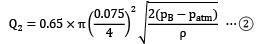Using equation ① and ②, we have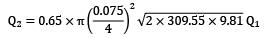= 0.224 Q1

Hence, Q = 1.224 Q1

Applying Bernoulli’s equation between A and C through the path ABC, we have,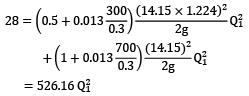which gives Q1 = 0.231 m3/s

Q2 = 0.224 × 0.231 = 0.052 m3/s

QUESTION: 17

A pipeline conveys 8.23 litre per second of water from an overhead tank to a building. The pipe is 2 km long and 0.15 m in diameter. It is desired to increase the discharge by 30% by installing another pipeline in parallel with this over half the length. Suggest a suitable diameter of the pipe to be installed. Is there any upper limit on discharge augmentation by this arrangement? (Take friction factor f = 0.03. )

Solution: The height H of the overhead tank above the building can be determined from the conditions with a single pipe.

d = 0.15 m

l = 2 km

f = 0.03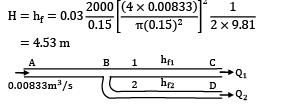In the new plan as shown in figure hf = 4.53 = hfAB + hfBC ⋯ ① again,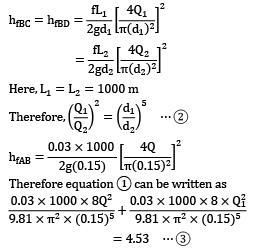In this case, Q = 1.3 × 0.00833 = 0.0108 m3/s Then, from equation ③, we get

Q12 = 0.00014 − (0.0108)2

which gives Q1 = 0.0048 m3/s From continuity,

Q2 = 0.0108 − 0.0048

= 0.006 m3/s

From equation ②, we have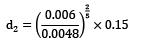?2 = 0.1?? ?

It can be observed from equation ③ that

Q12 = 0.00014 − Q2

or Q2 = 0.00014 − Q21

Now, Q will be maximum when Q1 will be minimum. For a physically possible situation, the minimum value of Q1 will be zero. Therefore, the maximum value of Q will be

Qmax = √0.00014 = 0.0118 m3/s which is 41.6% more than the initial value. The case (Q1 = 0, Q = 0.0118 m3/s) corresponds to a situation of an infinitely large branched pipe, i.e. d2 → ∞.

QUESTION: 18

A liquid of dynamic viscosity 0.04 Pa s and specific weight 8 kN⁄m3 is being pumped through a pipe of 1.25 cm diameter laid on slope as shown in Fig. For the pressures indicated, work out the direction and rate of flow (in ml/s) through the pipe.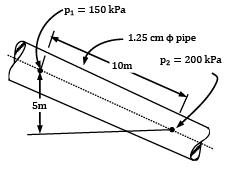Solution: Since the pipe is of uniform diameter, velocity head would be the same at the two sections and as such flow direction will be dictated by the values of the piezometric head. Taking a horizontal line passing through point 2 as the datum, then

p1 = p1 + wy1

= 150 + (8 × 5) = 190 kPa

p2 = p2 + wy2

= 200 + (8 × 0) = 200 kPa

Since p2 is greater then p1 , the direction of flow is from point 2 to point 1.

Using the Hagen–Poiseuille law,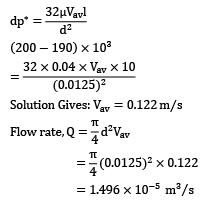QUESTION: 19

A pipe 10 cm in diameter and 1000 m long is used to pump oil of viscosity 3.5 poise and specific gravity 0.92 at the rate of 1200 litre/min. The first 300 m of the pipe is laid along the ground sloping upwards at 10° to the horizontal and the remaining pipe is laid to the ground sloping upwards at 15° to the horizontal. Determine the power (in kW) of the driving motor if the pump efficiency is 60 percent. Assume suitable data for friction coefficient f, if required.

Solution: Discharge, Q = 1200 litre⁄min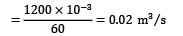Viscosity, μ = 8.5 poise = 8.5 × 0.1 = 0.85 N s⁄m

Flow velocity = discharge / area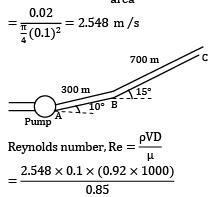= 275.78

Since the Reynolds number is less than 2000, the flow is laminar. For laminar flow, the friction coefficient is given by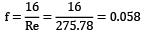Head loss due to friction in 1000 m length of pipe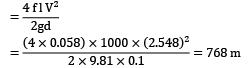The pressure to be developed by the pump can be worked out by applying Bernoulli’s equation between the pump outlet (suffix 1) and the end of the pipeline (suffix 2)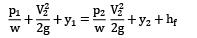With horizontal line through the pump center,

y1 = 0

and y2 = 300 sin 10° + 700 sin 15° = 233.25 m p2 = 0 as the pressure at the pipe exit is atmospheric Also V1 = V2

∴p1 / w = 233.25 + 768

= 1001.25 m of oil

Power required to develop this pressure

= w Q H

= (0.92 × 1000 × 9.81) × 0.02 × 1001.25

= 180729 Nm⁄s ≈ 180.73 kW

Power of driving motor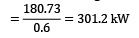QUESTION: 20

A circular pipe of radius R1 is placed concentrically inside another pipe of radius R2. If the flow in the annular space between the pipes is laminar. Find the r where maximum velocity occurs.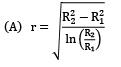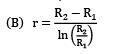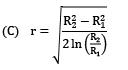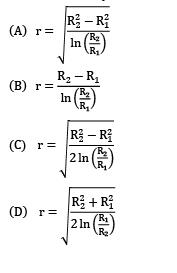Solution: Consider an annular element of radius r, thickness dr and length dx. Further let the velocity at radius r be u and pressure at two sections dx apart be p and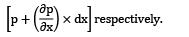Force on the element due to pressure difference is,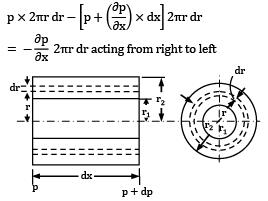Figure flow through an annular space Let shear stresses on the inner and outer surfaces of the element be τ and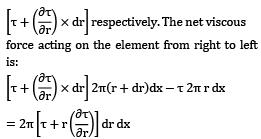Since the fluid is not accelerating (i.e., is moving with constant velocity), the sum of the pressure and viscous forces acting on the element must be zero. Thus: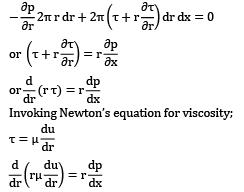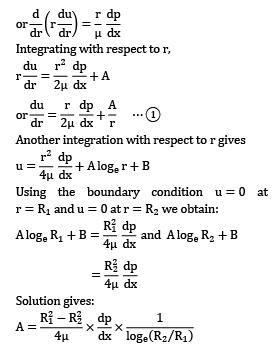Local velocity u would be maximum when